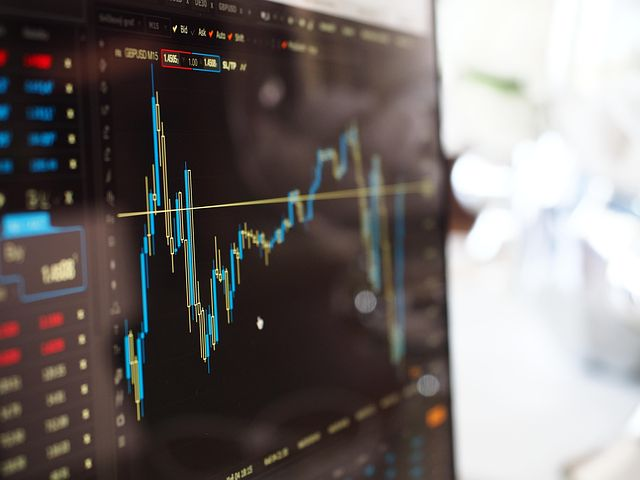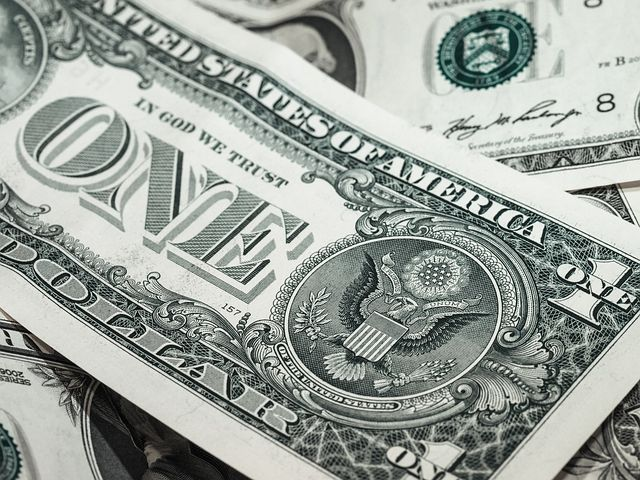# Future Value (FV) – Definition, Concept & Calculation

75 / 100

## Future Value – Definition, Concept & Calculation

Contents Preview

The future value (FV) is the value that a certain amount of money that we currently hold or that we decide to invest in a certain project will have in the future.### What is Future Value?

Future value is the future amount of an investment made today, which will grow over a period of time. The information provided by this method is useful because it allows you to calculate how much a company’s future wealth will be maximized, making it an important method for capital investment decisions.

The future value (FV) allows us to calculate how the value of the money that we currently have (today) will change considering the different investment alternatives that we have available. In order to calculate the future value, we need to know the value of our money at the current moment and the interest rate that will be applied in the coming periods.

Related Topic ~  Euro Zone (Euro Area) Definition, Features, Countries

The concept of future value is related to that of present value. The latter reflects the value that a flow of money that we will receive in the future would have today.

Future value is used to evaluate the best alternative as to what to do with our money today. Also to see how the value of money changes over time.### Concept of Future Value

The concept of future value seeks to reflect the fact that, if we decide to delay our current consumption, it will be for a prize, something worthwhile. In this way, we expect the future value to be greater than the present value of an amount of money that we currently have since a certain interest rate or return is applied to it.

Thus, for example, if today I decide to deposit money in a bank savings account, this amount will grow at the interest rate that the bank offers me.

### Relationship Between Present Value and Future Value

These are two sides of the same coin. They both reflect the value of the same money at different points in time. It is always better to have the money today, instead of waiting, unless we are paid interest for it. In the future value formula we can solve for the present value and vice versa.

Related Topic ~  Value at Risk (VAR) - Definition, Importance, Merits & Demerits

### Formula for Calculating the Future Value

The formula for calculating the future value depends on whether the interest applied is simple or compound.

• #### Simple interest formula

It occurs when the interest rate is applied only on the principal or initial amount, not on the interests that are earned over time. The formula is as follows:

FV = VP x (1 + rxn)

Where:

FV = Future Value

PV =  Present Value (the amount we invest today to earn interest)

r = simple interest rate

n = number of periods

Example: Suppose you invest \$ 1,000 in a savings account that offers a simple interest rate of 10%. What is the future value in the next two years?

FV = 1,000 x (1 + 10% x 2) = 1,200 euros (interest earned is 200)

• #### Compound interest formula

In this case, the interest rate is applied on the initial amount and also on the interest that is earned each period. The formula is as follows:

FV = PV x (1 + r) n

### Example of How to Calculate Future Value

Example: Suppose the bank now offers you a compound interest rate of 10% on savings. What is the future value in the next two years?

FV = 1,000 x (1 + 10%) 2  = 1,210 euros

Related Topic ~  Fair Value - Definition, Features & Calculations

This implies that the interest earned is 210. The first year the interest is 10% of 1,000 (100 euros), and the second year it is 10% of 1,100 (110 euros).